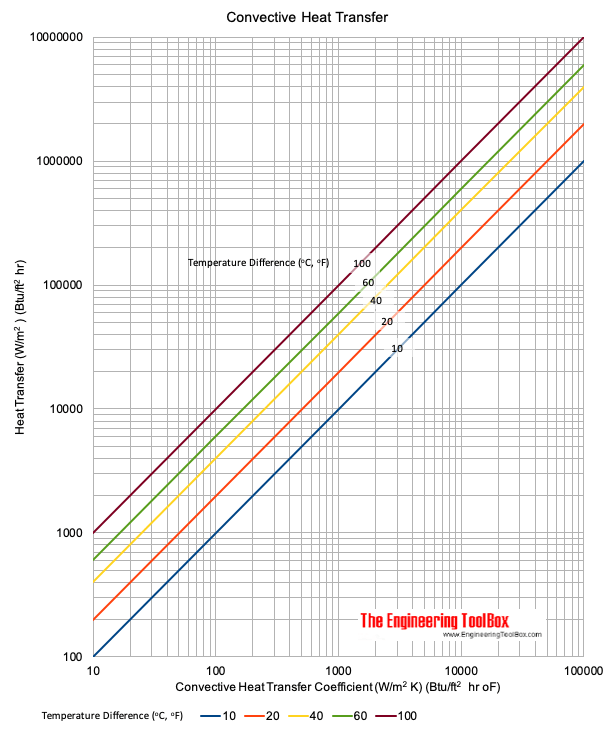## Convective Heat Transfer: How Do We Use It?

Vortex Tubes have been studied for decades, close to a century. These phenoms of physics and the theory behind them have been discussed on this blog before. Many customers gravitate toward Vortex Tubes when needing parts and processes cooled. The fact of the matter is there is still more to be discussed on how to correctly select the which product may be needed in your application. The reason being, area, temperatures, and air flow volumes play a large role in choosing the best product for cooling. The tendency is to say, well I need to cool this down as far as possible so I need the coldest air possible which leads to the assumption that a Vortex Tube will be the right solution. That isn’t always the best option and we are going to discuss how to best determine which will be needed for your application. The first step, is to call, chat, or email an Application Engineer so that we can learn about your application and assist with the implementation of the Vortex Tube or other cooling product for you. You may also want to try and take some initial readings of temperatures. The temperatures that would help to determine how much cooling is going to be needed are listed below:
• Part temperature
• Part dimensions
• Part material
• Ambient environment temperature
• Compressed air temperature
• Compressed air line size
• Amount of time desired to cool the part: Lastly desired temperature

With these bits of information, we use cooling equations to help determine what temperature and volume of air will best suit your needs to generate the cooling required. One of the equations we will sometimes use is the Forced or Assisted Convective Heat Transfer. Why do we use convective heat transfer rather than Natural Heat Transfer? Well, the air from EXAIR’s Intelligent Compressed Air Products® is always moving so it is a forced or assisted movement to the surface of the part. Thus, the need for Convective Heat Transfer.
Calculation of convection is shown below: q = hc A dT Where: q = Heat transferred per unit of time. (Watts, BTU/hr) A = Heat transfer area of the surface (m2 , ft2) hc= Convective heat transfer coefficient of the process (W/(m2°C), BTU/(ft2 h °F) dT = Temperature difference between the surface and the bulk fluid (compressed air in this case) (°C, °F)

The convective heat transfer coefficient for air flow is able to be approximated down to hc = 10.45 – v + 10 v1/2

Where: hc = Heat transfer coefficient (kCal/m2 h °C) v = relative speed between the surface of the object and the air (m/s)

This example is limited to velocities and there are different heat transfer methods, so this will give a ballpark calculation that will tell us if we have a shot at a providing a solution.  The chart below is also useful to see the Convective Heat Transfer, it can be a little tricky to read as the units for each axis are just enough to make you think of TRON light cycles. Rather than stare at this and try to find the hidden picture, contact an Application Engineer, we’ve got this figured out.1 – Engineering ToolBox, (2003). Convective Heat Transfer. [online] Available at: https://www.engineeringtoolbox.com/convective-heat-transfer-d_430.html [02/10/2021]